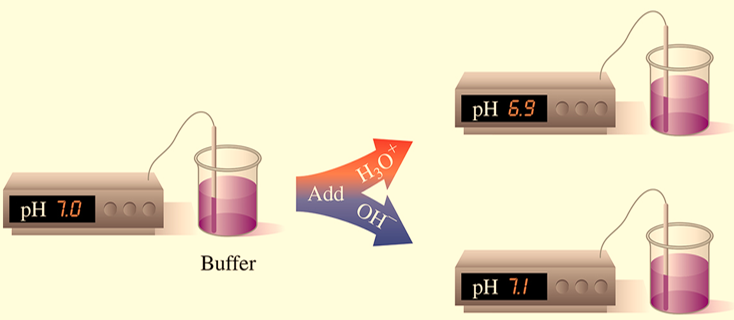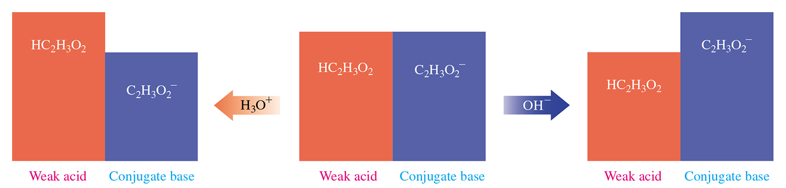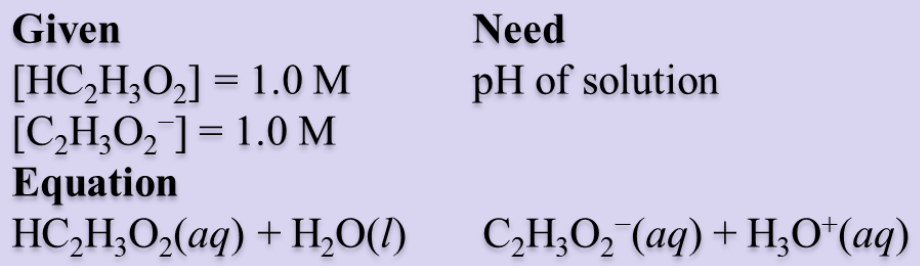# Buffer solution

Ionic Equilibrium: Buffer solution

Buffers are the solutions that withstand small changes in pH. Buffers can work only when small amounts of acid or alkali are added to them. They contain relatively large amounts of the weak acids (HA) and their conjugate base (A־), (or weak bases and their conjugate acids). Buffers can be prepared from conjugate acid-base pairs such as H2PO4/HPO42− andHPO42−/PO43−, HCO3/CO32−, or NH4+/NH3. The pH of the buffer solution will depend on the conjugate acid-base pair chosen.Fig: Illustration of a buffer solution

The functional capabilities of a buffer depend on a balance between a weak acid and its conjugate base. For example, in the buffer with acetic acid (HC2H3O2) and sodium acetate (NaC2H3O2), the salt produces acetate ions and sodium ions.

NaC2H3O2(aq) C2H3O2(aq) + Na+(aq)

The salt is added to provide a higher concentration of the conjugate base C2H3O2 than from the weak acid alone.

HC2H3O2(aq) + H2O(l) C2H3O2(aq) + H3O+(aq)

Large amount Large amount

If a small amount of base is added to this same buffer solution, it is neutralized by the acetic acid, HC2H3O2, which shifts the equilibrium in the direction of the products acetate ion and water. Equilibrium shifts in the direction of the products.

HC2H3O2(aq) + OH(aq) C2H3O2(aq) + H2O(l)

When a small amount of acid is added, the additional H3O+ combines with the acetate ion, C2H3O2, causing the equilibrium to shift in the direction of the reactants, acetic acid, and water. The acetic acid produced in this reaction contributes to the available weak acid. Equilibrium shifts in the direction of the reactants.

HC2H3O2(aq) + H2O(l) C2H3O2 (aq) + H3O+(aq)Fig : Working of a buffer system.

The buffer described in the figure above contains about equal concentrations of acetic acid (HC2H3O2) and its conjugate base, acetate ion (C2H3O2) in an equilibrium state. When H3O+is added to the buffer, it reacts with the salt, C2H3O2. Whereas, the addition of OH neutralizes the acid HC2H3O2. The pH of the solution is maintained if the added amounts of acid or base are small compared to the concentrations of the buffer components. The buffering capacity depends on concentrations of a weak acid or a weak base and their common ions. The effective pH buffering range is close to the pKa 1 unit. A buffer works optimally at mid-range, where the amount of undissociated acid and its conjugate base are equal and the pH=pKa. In this range, the acid and the salt are at the same concentration.

Consider the following numerical problem where the Ka for acetic acid, HC2H3O2, is 1.8 × 10–5. What is the pH of a buffer prepared with 1.0 M HC2H3O2 and 1.0 M C2H3O2?

## Analyze The ProblemFigure 3:

Step 1 is to state the given and needed quantities.

Step 2 is to write the acid ionization constant and rearranging for [H3O+]. Kaexpression from this reaction gives [H3O+], and we can obtain the ratio of the acetic acid/acetate buffer and calculate the pH.Solving for H3O+ givesStep 3 is to substitute [HA] and [A] into the Ka expression and then calculate pH.